# Identify the odd one out using direction, position and number

In this worksheet, students will identify an image that does not fit in with the rest of the group. The focus will be on direction, position and number, so students will need to look at where shapes are, which direction they are facing, and how many there are.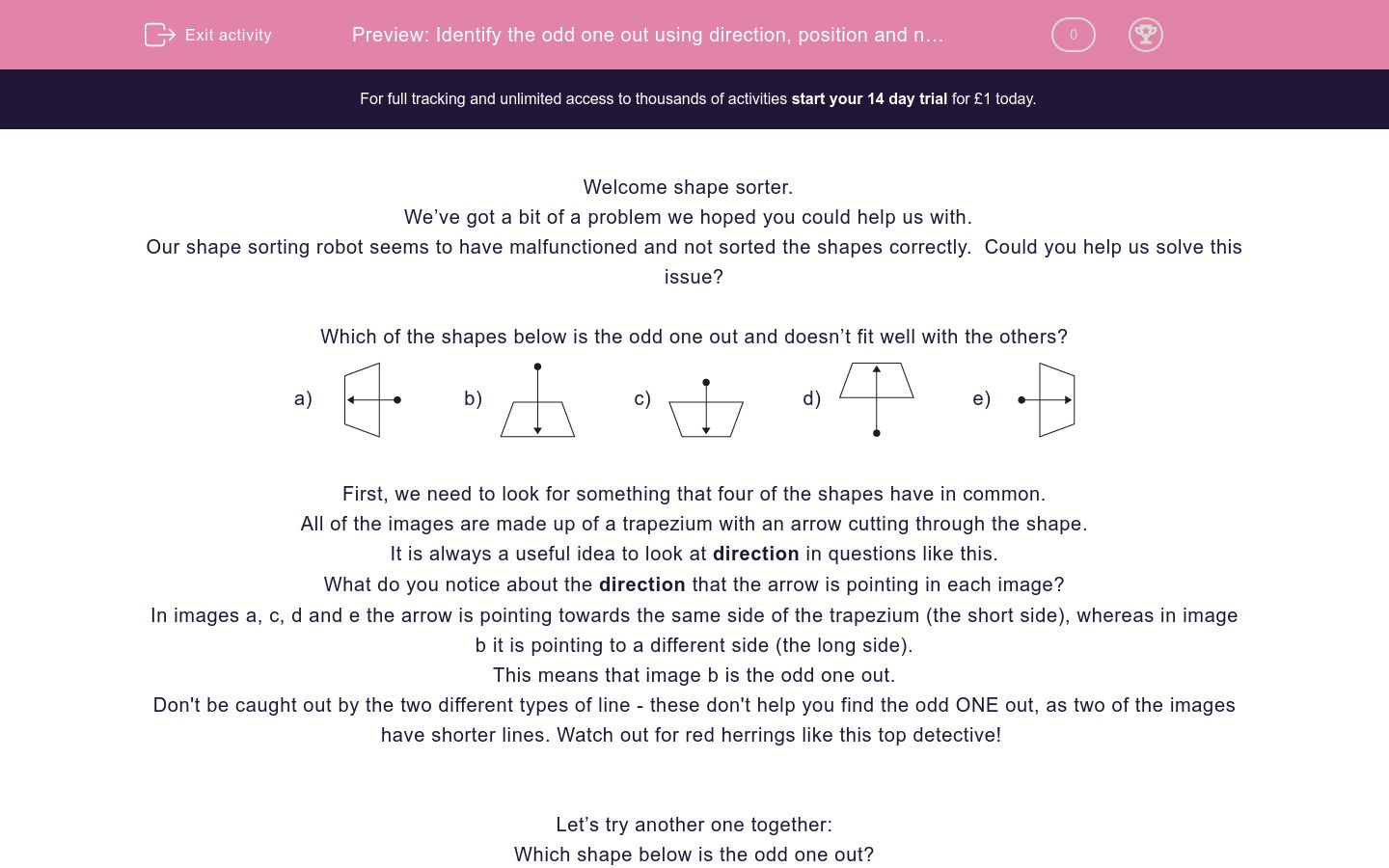Key stage:  KS 2

Curriculum topic:   Spatial and Non-Verbal Reasoning

Curriculum subtopic:   Odd One Out

Difficulty level:### QUESTION 1 of 10

Welcome shape sorter.

We’ve got a bit of a problem we hoped you could help us with.

Our shape sorting robot seems to have malfunctioned and not sorted the shapes correctly.  Could you help us solve this issue?

Which of the shapes below is the odd one out and doesn’t fit well with the others?

a)b)c)d)e)First, we need to look for something that four of the shapes have in common.

All of the images are made up of a trapezium with an arrow cutting through the shape.

It is always a useful idea to look at direction in questions like this.

What do you notice about the direction that the arrow is pointing in each image?

In images a, c, d and e the arrow is pointing towards the same side of the trapezium (the short side), whereas in image b it is pointing to a different side (the long side).

This means that image b is the odd one out.

Don't be caught out by the two different types of line - these don't help you find the odd ONE out, as two of the images have shorter lines. Watch out for red herrings like this top detective!

Let’s try another one together:

Which shape below is the odd one out?

a)b)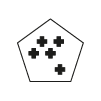c)d)e)First, we need to look for something that four of the shapes have in common.

In questions like this, counting is really important. What could we count?

We have two things to count here: the number of mini shapes and the number of sides of the main shape.

Image a is made up of a 3-sided shape with 3 crosses inside.

Image b is a 5-sided shape with 5 crosses inside.

Image c is a 4-sided shape with 4 crosses inside.

Image d is a 6-sided shape with 5 crosses inside.

Image e is a 4-sided shape with 4 crosses inside.

This means that image d is the odd one out as the number of sides of the main shape does not match the number of mini shapes inside, unlike the other options.

It’s now your turn to identify the odd image.

Remember to look at the direction and position of shapes within the image and always see if there is something you can count.

Good luck shape sorter!

Which of the images below is the odd one out?

a)b)c)d)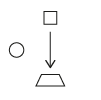e)Which of the images below is the odd one out?

a)b)c)d)e)Which of the images below is the odd one out?

a)b)c)d)e)Which of the images below is the odd one out?

a)b)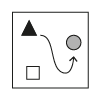c)d)e)Which of the images below is the odd one out?

a)b)c)d)e)Which of the images below is the odd one out?

a)b)c)d)e)Which of the images below is the odd one out?

a)b)c)d)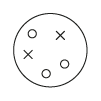e)Which of the images below is the odd one out?

a)b)c)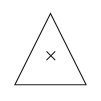d)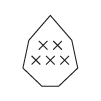e)Which of the images below is the odd one out?

a)b)c)d)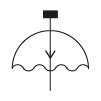e)Which of the images below is the odd one out?

a)b)c)d)e)• Question 1

Which of the images below is the odd one out?

d)EDDIE SAYS
This was a challenging one. Normally when we see an arrow, we look at where it is pointing TO. However, the arrows are all pointing at different white shapes here. In this question, it is important to see where the arrow is coming FROM. In options a, b, c and e the arrow is coming from a black shape, but in option d it is coming from a white shape. This makes option the odd one out.
• Question 2

Which of the images below is the odd one out?

b)EDDIE SAYS
This is a number relationship question. In four of the images, there is a connection between the number of crosses and the number of sides of the main shape. Can you spot the relationship? Options a, c, d and e all have a matching number of sides and crosses. For example, option a has 3 sides and 3 crosses, and option c has 6 sides and 6 crosses. However, option b has 4 sides but a sneaky 5 crosses, making it the odd one out.
• Question 3

Which of the images below is the odd one out?

c)EDDIE SAYS
Here's another number relationship question. There is a connection between the number of circles and the number of sides of each shape. Can you spot how they are linked top detective? In options a, b, d and e there are the same number of circles as there are sides to the shape. For example, in option a there are 3 sides and 3 circles. This pattern doesn't work for option c, as it has 4 sides but only 3 circles. This makes option c the odd one out.
• Question 4

Which of the images below is the odd one out?

e)EDDIE SAYS
Don't worry if you missed the POINT in this question detective, there is more than one clue to consider here. Let's look at where the arrow is coming from AND where it is going. In options a, b, c and d the arrow is coming from a black shape but pointing to a grey shape. In option e the arrow is coming from a black shape but pointing to a white shape instead. This makes option e the odd one out.
• Question 5

Which of the images below is the odd one out?

e)EDDIE SAYS
This is a number-themed question so get your magnifying glass on to count these! In the staircase images in a, b, c and d, there is one less step each time. For example in option a, there is 1 step at the top, then 2, then 3. Similarly in option b, there is 1 step, then 2, then 3, then 4, then 5. The number of steps is increasing by 1 each time. In option e, the stairs start off correctly but there is a sudden jump from 3 to 5 steps. These stairs would trip someone up, but don't let them get you here! This makes option e the odd one out.
• Question 6

Which of the images below is the odd one out?

b)EDDIE SAYS
Here there is a relationship between the number of sides of the outside shape and the number of sides of the inside shape. It is not a mirror-relationship so they are not the same. For example, in option a there is a 4-sided shape with a 3-sided shape inside. In option c, there is a 5-sided shape with a 4-sided shape inside. The pattern is that the middle shape has one less side than the outer shape. This doesn't work for option b, as it has a 4-sided shape with a 5-sided shape inside. For this reason, option b is the odd one out.
• Question 7

Which of the images below is the odd one out?

b)EDDIE SAYS
We have a few options of what to count here. We have white circles, shaded circles and crosses present. In options a, c, d and e there are 2 crosses and 3 circles. The shading of the circles changes and tries to catch us out, but really it doesn't matter, so it is red herring! Option b has an extra circle, making it the odd one out.
• Question 8

Which of the images below is the odd one out?

e)EDDIE SAYS
This was a challenging counting question! There is a relationship between the number of sides of each shape and the number of crosses inside each. Option a has 6 sides and 4 crosses. Option b has 4 sides and 2 crosses. Option c has 3 sides and 1 cross. Option d has 7 sides and 5 crosses. So there are 2 less crosses than the number of sides in each of these cases. Option e doesn't fit this rule as it has 4 sides but 3 crosses. So option e is the odd one out.
• Question 9

Which of the images below is the odd one out?

c)EDDIE SAYS
These shapes look like umbrellas - let's hope it is raining clues on how to solve this one! This is a direction-themed question. Take a look at where the arrow is pointing from and to. In options a, b, d and e the arrowhead is pointing in the direction of the wavy line, whichever direction this is facing. In option c it is pointing to the curved line of the umbrella shape. This makes option c the odd one out.
• Question 10

Which of the images below is the odd one out?

d)EDDIE SAYS
Here is your final direction-themed question. You'll be an expert at giving and receiving directions with all of this practise! Options a, b, c and e are all pointing in an anti-clockwise direction, whereas option d is pointing clockwise. This makes option d the odd one out. Hopefully, you have learnt that a good understanding of clockwise and anti-clockwise is another important skill for a top detective to have!
---- OR ----

Sign up for a £1 trial so you can track and measure your child's progress on this activity.

### What is EdPlace?

We're your National Curriculum aligned online education content provider helping each child succeed in English, maths and science from year 1 to GCSE. With an EdPlace account you’ll be able to track and measure progress, helping each child achieve their best. We build confidence and attainment by personalising each child’s learning at a level that suits them.

Get started